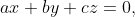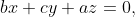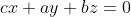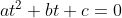# Basis maths-linear algebra-Q1

+1 vote

If and the system of equationshas a non trivial solution then the roots of the quadratic equationare

(A). Both Roots are Real and Positive

(B). Both Roots are Real and Negative

(C). Complex But Not Real

(D). One Root is Positive and One is Negativeasked May 15
reshown May 16

A   homogeneous system of linear equations has a unique solution (the trivial solutionif and only if its determinant is non-zeroIf this determinant is zero, then the system has an infinite number of solutions.

So,  the system of equationshas a non trivial solution, it means that :

So,

So

We know that

Since , So,

this means that either

cannot be zero because:

As , The given value cannot be zero.

This means (a + b + c ) has to be zero.

So, , and     , so,

So, .

The quadratic equationhas real solutions iff

Since, a,c are of different signs, so, . Hence, the roots of the equationare real.

We know that if X,Y are roots ofthen

Since a,c are of different signs, so, XY is negative, so, Both roots are of different signs.answered Jun 12 by (112,760 points)answered Jun 12 by (7,690 points)